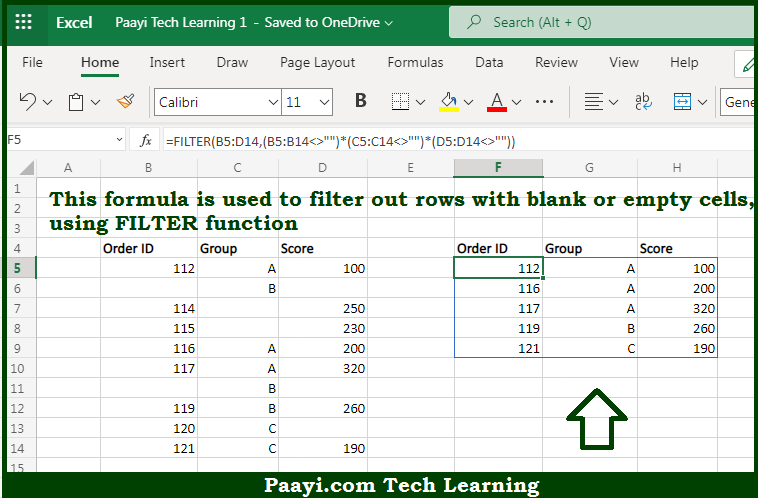# Learn How to Filter Exclude Blank Values in Microsoft Excel

Written by | 0 Comments | 665 Views

In this article, you will learn how to evaluate things in Dynamic Arrays with formulas in Microsoft Excel using a single/combination(s) of functions. You will also know How toFilter Exclude Blank Values and see the generic formula.

Learn How to Filter Exclude Blank Values in Microsoft Excel

The main purpose of this formula is to filter out rows with blank or empty cells. Here we will learn how to filter excluding the blank rows in the given data range in the workbook in Microsoft Excel. That implies, with the help of a formula based on the FILTER function you can able to filter out rows with blank or empty cells. So, with the help of this formula, you can able to filter excluding the blank rows in the given data range in the workbook in Microsoft Excel.

General Formula to Filter Exclude Blank Values

=FILTER(data,(rng1<>"")*(rng2<>"")*(rng3<>""))

The Explanation for the Filter Exclude Blank ValuesSo we know that with the help of the given formula above you can able to filter out rows with blank or empty cells. Here we will learn how to filter excluding the blank rows in the given data range in the workbook in Microsoft Excel. As we know that the  FILTER function is made to extract data matching one or more criteria. Here, we want to apply criteria that require all three columns in the source data which are - Name, Group, and Rooms. That implies, if a row is missing any of these values, we want to exclude that row from the output. That is why we use three Boolean expressions operating on arrays. So, with the help of this formula, you can able to filter out rows with blank or empty cells. Here we will learn how to filter excluding the blank rows in the given data range in the workbook in Microsoft Excel.